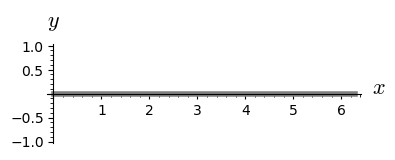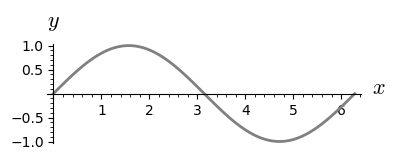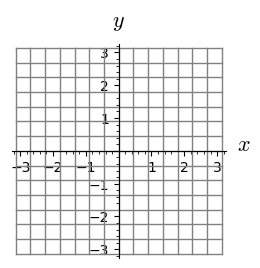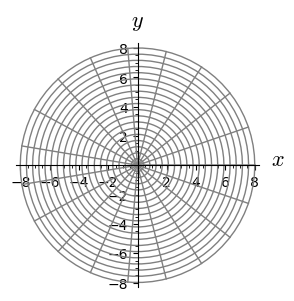# 3. Function graph as a manifold¶

This notebook is part of the Introduction to manifolds in SageMath by Andrzej Chrzeszczyk (Jan Kochanowski University of Kielce, Poland).

In :
version()

Out:
'SageMath version 9.6, Release Date: 2022-05-15'

The graph $$Γ(f)=\{(x, f(x))\in R^{m+n}: x ∈ U \},$$

for a smooth function $f : U → R^{m}$ and an open subset $U\subset R^n$ is a simple example of a smooth manifold having an atlas with a single chart

$$(Γ( f ), φ ),\quad \phi: (x, f (x)) \to x,\quad x\in U.$$

### One-dimensional function graph as a manifold¶

Since we don't use any predefined charts or transitions in this case, we can use general manifold (not the Euclidean space as previously).

Example 3.1

Here we use an open interval $(0,2\pi)$:

In :
J = manifolds.OpenInterval(0, 2*pi)  # open interval J as manifold
c.<t>=J.chart()                      # chart on J


First we plot $U=(0,2\pi)$ as a subset of $R^2$ defined as $x(t)=t,\quad y(t)=0.$

In :
R2 = Manifold(2, 'R^2')             # manifold R^2
X.<x,y> = R2.chart()                # chart on R^2
# F1: J -> R^2 :
F1 = J.continuous_map(R2,{(c, X):[t,0]}, name='F1')
# plot c
p1=c.plot(X,mapping=F1,thickness=4,color='grey')
p1.show(figsize=[4,3])              # show plotAnd next the image of $U$ under sinus function as a subset of $R^2$.

In :
                                    # continuous map x=t,y=sin(t):
F2 = J.continuous_map(R2, {(c, X): [t,sin(t)]}, name='F2')
p=c.plot(X,mapping=F2,color='grey',thickness=2) # plot image of J
p.show(figsize=[4,3])                           # under F2Example 3.2

Below, we map the same open interval into $R^3$.

In :
# continuation
po1={'thickness':5,'color':'darkblue'}         # param.
po2={'fontsize':20,'color':'black'}

ax =line3d([(0,0,0), (1+0.15,0,0)], **po1)     # axes
ax+=line3d([(0,0,0), (0,1+0.15,0)], **po1)
ax+=line3d([(0,0,0), (0,0,6.28+0.15)], **po1)
ax+=text3d("x",(1.25,0,0),**po2)
ax+=text3d("y",(0,1.25,0),**po2)
ax+=text3d("z",(0.,0.,6.9),**po2)

In :
J=manifolds.OpenInterval(0,2*pi)  # open interval as manifold
c.<t>=J.chart()                   # chart on J
R3 = Manifold(3, 'R^3')           # manifold R^3
X.<x,y,z> = R3.chart()            # chart on R^3
# F: J -> R^3:
F=J.continuous_map(R3,{(c,X):[cos(3*t),sin(3*t),t]},name='F')
p=c.plot(X,mapping=F,color='grey',thickness=3,
plot_points=200,label_axes=False)  # plot image of J
(p+ax).show(aspect_ratio=[1,1,0.3],frame=False)  # show plot


### Two-dimensional function graph as a manifold¶

Example 3.3

Now let $U$ be an open rectangle $(-\pi,\pi)\times (-\pi,\pi)$ in $R^2$.

In :
R2 = Manifold(2, 'R^2')                  # manifold R^2
c_xy.<x,y> = R2.chart()                  # chart on R^2
U = R2.open_subset('U',                  # open subset of R^2
coord_def={c_xy: [x>-pi,x<pi,y>-pi,y<pi]})
c_U.<x,y>=U.chart()                      # chart on U


First we plot the coordinate lines in the set $(-\pi,\pi)\times(-\pi,\pi)$.

In :
p=c_U.plot(number_values={x:15,y:15},color='grey')  # plot
p.show(figsize=4)                        # coordinate lines on UThe graph of the function $f(x,y)=\cos(\sqrt{x^2+y^2})$ as a subset of $R^3$:

In :
R3 = Manifold(3, 'R^3')                     # manifold R^3
c_XYZ.<X,Y,Z> = R3.chart()                  # chart on R^3
F = U.continuous_map(R3,                    # F: U -> R^3
{(c_U, c_XYZ): [x,y,cos(sqrt(x^2+y^2))]}, name='F')
# plot the image of coordinate lines in  U:
p1=c_U.plot(c_XYZ,mapping=F,number_values={x:15,y:15},
color='black',thickness=2,label_axes=False)
s=plot3d(cos(sqrt(x^2+y^2)),(x,-pi,pi),(y,-pi,pi),
color='lightgrey',opacity=0.7)     # plot  image of U
(p1+s).rotateZ(-pi/6).show(frame=False,aspect_ratio=[1,1,1.8])
# show plot


Example 3.4

Now we show a similar example using polar coordinates. We need two charts in this example. If we don't use the Euclidean space, we need to define both Cartesian and polar coordinates and also the transition map

$$\ \ x=r\cos\phi,\ \ y=r\sin\phi.$$

First we plot the coordinate lines $r,\phi$:

In :
reset()
R2 = Manifold(2, 'R^2')                  # manifold R^2
c_cart.<x,y> = R2.chart()                # Cartesian coordinates
# the complement of the segment y=0 and x>=0:
U = R2.open_subset('U', coord_def={c_cart: (y!=0, x<0)})
c_pol.<r,ph> = U.chart(r'r:(0,+oo) ph:(0,2*pi):\phi') # polar coord.
# transition map:
pol_to_cart = c_pol.transition_map(c_cart, [r*cos(ph), r*sin(ph)])
# plot coordinate lines
g = c_pol.plot(c_cart,number_values={r:20,ph:20},color='grey')
g.show(figsize=[3,3])                    # show plotAnd next the image of these lines as a subset of $R^3$:

In :
# continuation
R3 = Manifold(3, 'R^3')                  # manifold R^3
c_XYZ.<X,Y,Z> = R3.chart()               # chart on R^3
F = U.continuous_map(R3,                 # F: U->R^3
{(c_pol, c_XYZ): [r*cos(ph),r*sin(ph),cos(r)]}, name='F')
# plot images of coordinate lines in U:
p=c_pol.plot(c_XYZ,mapping=F,number_values={r:20,ph:20},
color='black',thickness=1,label_axes=False)
# plot image of U:
s=parametric_plot3d([r*cos(ph),r*sin(ph),cos(r)],
(ph,0,2*pi),(r,0,8.1),color='lightgrey',opacity=0.5)
(p+s).show(aspect_ratio=[1,1,4],frame=False)  # show plot


### Three dimensional function graph as a manifold¶

Example 3.5

First we show the coordinate lines $x,y,z$ in the three dimensional rectangle:

In :
M = Manifold(3, 'M')                   # manifold M
c_xyz.<x,y,z> = M.chart()              # coordinates x,y,z
p1=c_xyz.plot(ranges={x:(0.1,1),y:(0.1,1),z:(0.1,1)},
number_values={x:4,y:4,z:4},thickness=2,
color='grey',label_axes=False)     # plot coordinate lines
p1.show(frame=False)                   # show plot
# adjust manually the figure after plot
# (figsize does not work in 3d)